#### Surgical: Institutional ranking

This example considers mortality rates in 12 hospitals performing cardiac surgery in babies. The data are shown below.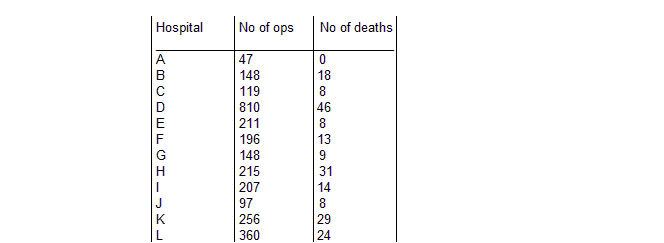The number of deaths ri for hospital i are modelled as a binary response variable with `true' failure probability pi:

ri ~ Binomial(pi, ni)

We first assume that the true failure probabilities are independent (i.e.fixed effects) for each hospital. This is equivalent to assuming a standard non-informative prior distribution for the pi's, namely:

pi ~ Beta(1.0, 1.0)

Graphical model for fixed effects surgical example: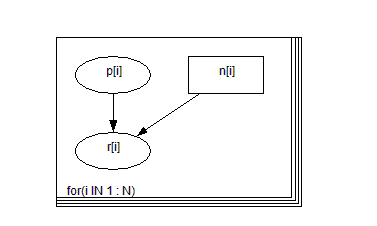BUGS language for fixed effects surgical model:

model
{
for( i in 1 : N ) {
p[i] ~ dbeta(1.0, 1.0)
r[i] ~ dbin(p[i], n[i])
}
}

##### Data
``` list(n = c(47, 148, 119, 810,   211, 196, 148, 215, 207, 97, 256, 360),   r = c(0, 18, 8, 46, 8, 13, 9,   31, 14, 8, 29, 24),   N = 12) ```
##### Inits for chain 1
```    list(p = c(0.1, 0.1, 0.1, 0.1,   0.1, 0.1, 0.1, 0.1, 0.1, 0.1, 0.1, 0.1)) ```

##### Inits for chain 2
```    list(p = c(0.5, 0.5, 0.5, 0.5,   0.5, 0.5, 0.5, 0.5, 0.5, 0.5, 0.5, 0.5)) ```
A more realistic model for the surgical data is to assume that the failure rates across hospitals are similar in some way. This is equivalent to specifying a random effects model for the true failure probabilities pi as follows:

logit(pi) = bi

bi ~ Normal(μ, τ)

Standard non-informative priors are then specified for the population mean (logit) probability of failure, μ, and precision, τ.
Graphical model for random effects surgical example: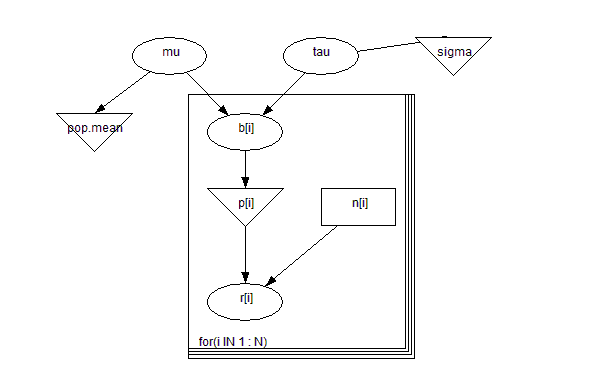BUGS language for random effects surgical model:

model
{
for( i in 1 : N ) {
b[i] ~ dnorm(mu,tau)
r[i] ~ dbin(p[i],n[i])
logit(p[i]) <- b[i]
}
pop.mean <- exp(mu) / (1 + exp(mu))
mu ~ dnorm(0.0,1.0E-6)
sigma <- 1 / sqrt(tau)
tau ~ dgamma(0.001,0.001)
}

##### Data
``` list(n = c(47, 148, 119, 810,   211, 196, 148, 215, 207, 97, 256, 360),   r = c(0, 18, 8, 46, 8, 13, 9,   31, 14, 8, 29, 24),   N = 12) ```
##### Inits for chain 1
``` list(b = c(   0.1,   0.1,   0.1,   0.1,   0.1,   0.1,   0.1,   0.1,   0.1,   0.1,   0.1,   0.1),    tau = 1, mu = 0) ```

##### Inits for chain 2
``` list(b = c(   0.5,   0.5,   0.5,   0.5,   0.5,   0.5,   0.5,   0.5,   0.5,   0.5,   0.5,   0.5),    tau = 0.1, mu = 1.0) ```
Results for independent model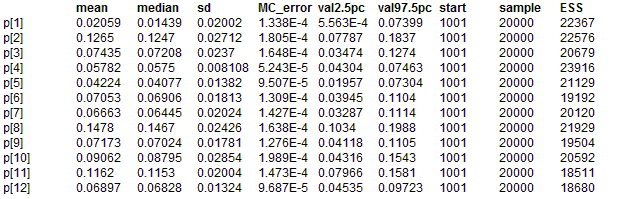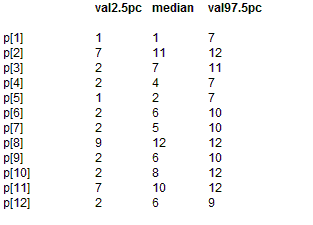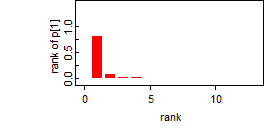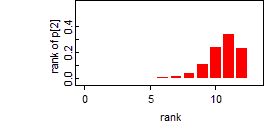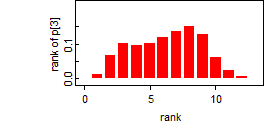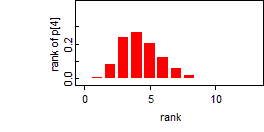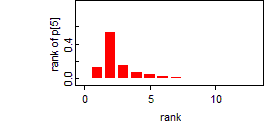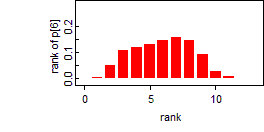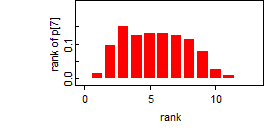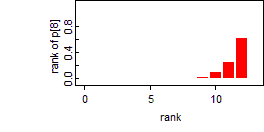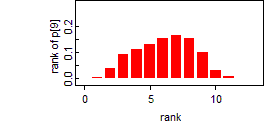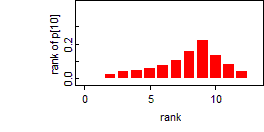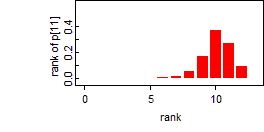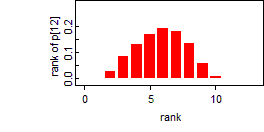Results for random effects model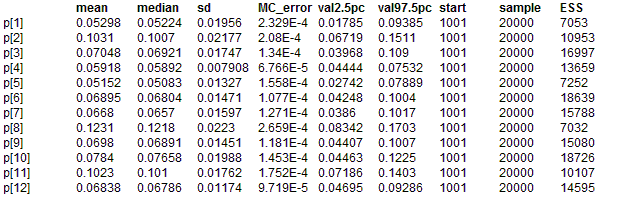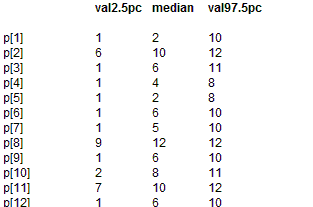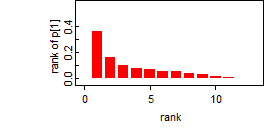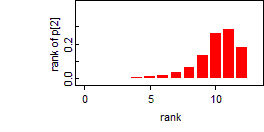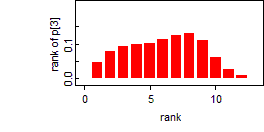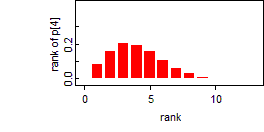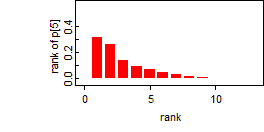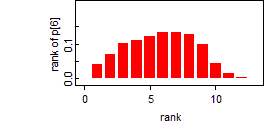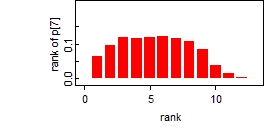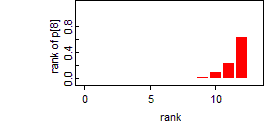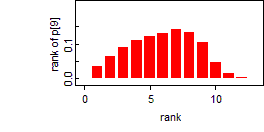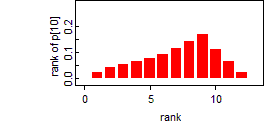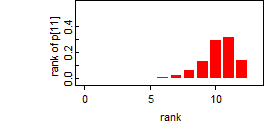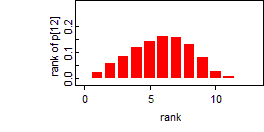A particular strength of the Markov chain Monte Carlo (Gibbs sampling) approach implemented in BUGS is the ability to make inferences on arbitrary functions of unknown model parameters. For example, we may compute the rank probabilty of failure for each hospital at each iteration. This yields a sample from the posterior distribution of the ranks.

The figures below show the posterior ranks for the estimated surgical mortality rate in each hospital for the random effect models. These are obtained by setting the rank monitor for variable p (select the "Rank" option from the "Statistics" menu) after the burn-in phase, and then selecting the "histogram" option from this menu after a further 10000 updates. These distributions illustrate the considerable uncertainty associated with 'league tables': there are only 2 hospitals (H and K) whose intervals exclude the median rank and none whose intervals fall completely within the lower or upper quartiles.